Choose Language Hide Translation Bar
Turtle Graphics

Turtle graphics is ancient. Tell a turtle to walk forward 10 steps, turn right 90 degrees, repeat 4 times... Give the turtle a pen and you've drawn a square. Here's a set of drawing routines that wrap the JSL drawing functions to make a turtle. First the routines, then examples.

``````Delete Namespaces( "turtle" );
turtle = New Namespace( "turtle" );

/*
The turtle graphics idea has a lot of history and a lot of different implementations.
This set of functions is incomplete and just for fun and might be useful to someone.
The functions ending in ABS make an absolute change and the REL functions a relative
change. Except drawRel() is named walk(); mostly the turtle walks in the current
direction. turnRel() will add a few degrees to the current direction and turnAbs()
sets an absolute direction. Scaling is similar; scaleRel(.5) makes a subsequent walk(1)
only half as far as before the scale. scaleRel(2) will put it back for the next walk().
And, there is no moveRel, just use walk with a pen size of 0.
The path (begin,add,fill,outline) functions were added late for the last example and
have a comment on how they might be extended.
*/

turtle:init = Function( {}, // each refresh needs to start with the same initial conditions
turtle:scaleFactor = 1;
turtle:angleDegrees = 0;
turtle:x = 0;
turtle:y = 0;
);

turtle:turnAbs = Function( {angle},
turtle:angleDegrees = angle
);

turtle:turnRel = Function( {degrees},
turtle:angleDegrees += degrees;
turtle:angleDegrees = Mod( 360 + turtle:angleDegrees, 360 );
);

turtle:scaleAbs = Function( {factor},
turtle:scaleFactor = factor
);

turtle:scaleRel = Function( {factor},
turtle:scaleFactor *= factor
);

turtle:moveAbs = Function( {x, y},
turtle:x = x;
turtle:y = y;
);

turtle:drawAbs = Function( {x, y, color = "black", thick = 1},
Pen Color( color );
Pen Size( thick );
Line( {turtle:x, turtle:y}, {x, y} );
turtle:x = x;
turtle:y = y;
);

turtle:rectAbs = Function( {x1, y1, x2, y2, color = "black"},
Fill Color( color );
Rect( x1, y1, x2, y2, 1 );
);

turtle:beginPath = Function( {},
turtle:CollectPoly = {{}};
turtle:CollectPoly = turtle:x;
turtle:CollectPoly = turtle:y;
turtle:CollectPoly = 1; // move

);

a = Pi() * turtle:angleDegrees / 180;
xnew = turtle:x + Cos( a ) * distance * turtle:scaleFactor;
ynew = turtle:y + Sin( a ) * distance * turtle:scaleFactor;
turtle:x = xnew;
turtle:y = ynew;
turtle:CollectPoly[N Items( turtle:CollectPoly ) + 1] = Eval List( {xnew, ynew, 2} );// draw
);

turtle:fillPath = Function( {color}, {temp},
Fill Color( color );
temp = Matrix( turtle:CollectPoly );
// if you think the path should be replayed at the
// current scale/rotate + pos, apply them here, and
// in outlinePath below. The idea would be to create
// the path at scale 1, around the origin, then use
// the origin as the center of rotation. Needs a bit
// more thought. For now, however, the paths are
// created, used, discarded and the absolute coords
// in a path will just overstrike if reused.
Path( temp, 1 );
);

turtle:outlinePath = Function( {color}, {temp},
Pen Color( color );
temp = Matrix( turtle:CollectPoly );
Path( temp, 0 );
);

// this is the heart of "turtle graphics" -- walk a distance in the
// current direction. If the pen thickness is > 0, draw a line.
turtle:walk = Function( {distance, color = "black", thick = 1}, {xnew, ynew, a},
a = Pi() * turtle:angleDegrees / 180;
xnew = turtle:x + Cos( a ) * distance * turtle:scaleFactor;
ynew = turtle:y + Sin( a ) * distance * turtle:scaleFactor;
If( thick > 0,
Pen Color( color );
Pen Size( thick );
Line( {turtle:x, turtle:y}, {xnew, ynew} );
);
turtle:x = xnew;
turtle:y = ynew;
);

// write a message at the current location
turtle:say = Function( {message, color = "black", points = 12},
Text Color( color );
If( points * turtle:scaleFactor >= 2, // textsize() gets unhappy with very small sizes
Text Size( points * turtle:scaleFactor );
Text( {turtle:x, turtle:y}, message );
);
);

// draw a marker at the current location
turtle:mark = Function( {color = "black"},
// 12 is a filled circle. if you need to control the size, either
// specify the markersize or use a filled pie. There can only be one
// markersize for the graph, and the filled pies may not keep their aspect.
Marker( Combine States( Color State( color ), Marker State( 12 ) ), {turtle:x, turtle:y} )
);

// tell the turtle to run a program and make a graph
turtle:run = Function( {title, program, bc = "white", xpixels = 900, ypixels = 900, xmin = -100, xmax = 100, ymin = -100, ymax = 100},
{},
// eval(evalexpr(...expr(nameexpr(...)))) builds the
// expression into the graph, freezing it. This means
// a second graph with a different expression can coexist.
// it isn't standalone though, because it still needs the turtle namespace.
Eval(
Eval Expr(
New Window( title,
Graph Box(
framesize( xpixels, ypixels ),
X Scale( xmin, xmax ),
Y Scale( ymin, ymax ),
turtle:init();
Expr( Name Expr( program ) );,
<<backgroundcolor( bc )
)
)
)
)
);``````

Spiral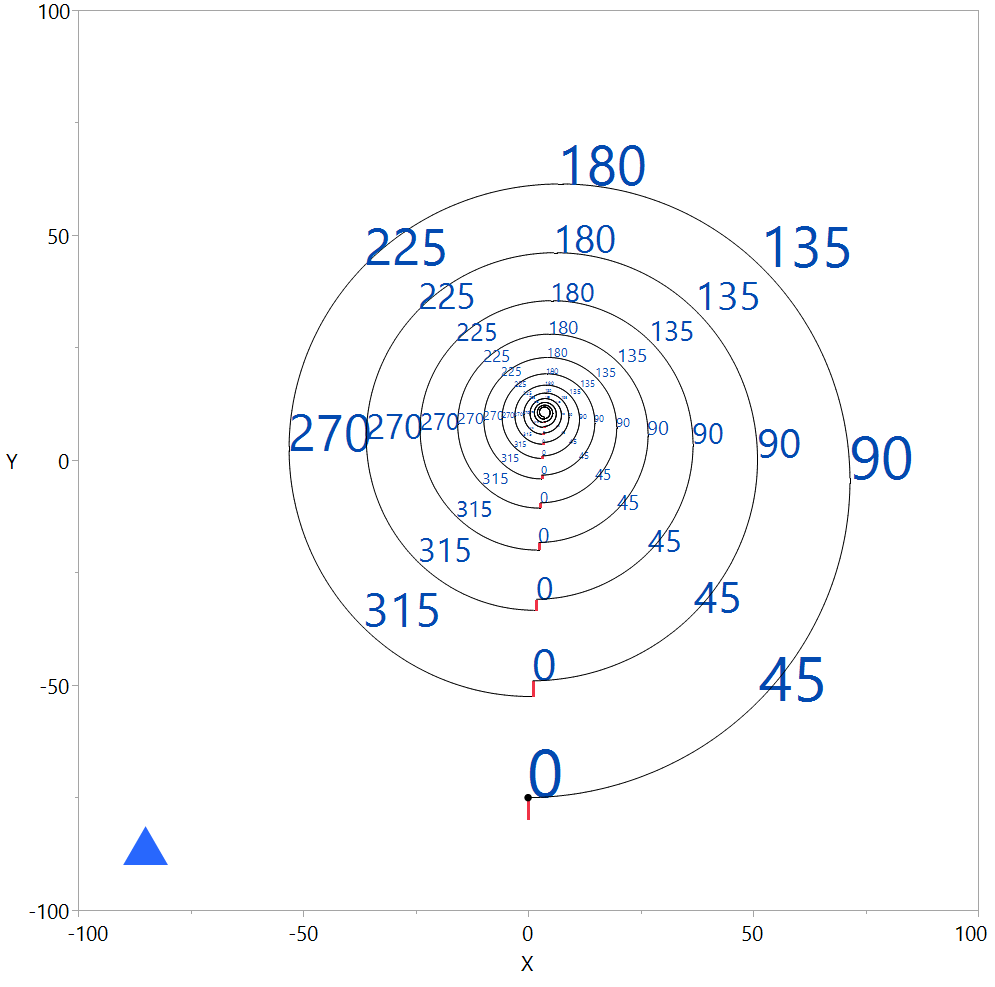Spiral example

``````turtle:run(
"Quick spiral test",
Expr(
Local( {i, j},
turtle:moveabs( -90, -90 );
turtle:beginpath(); // triangle at bottom left is a filled path...
turtle:addpath( 10 ); // move forward 10 at current angle
turtle:turnRel( 120 ); // spin 120 degrees
turtle:turnRel( 120 );
turtle:turnRel( 120 ); // back to zero angle
turtle:fillPath( "blue" ); // actually draws the stored path
// begin the spiral
turtle:moveAbs( 0, -80 ); // start at an empirically chosen location
For( j = 1, j <= 12, j += 1, // make several shrinking instances
turtle:turnRel( 90 ); // crosswise to current direction
turtle:walk( 5, "red", 3 ); // 5 gets scaled smaller and smaller (.999 below), pen width 3 does not shrink
turtle:turnRel( -90 ); // back to current direction
For( i = 0, i < 360, i += 1, // make a full loop in 1 degree steps
If( Mod( turtle:angleDegrees, 45 ) == 0, // mark the 45 degree points
If( turtle:angleDegrees == 0 & turtle:scaleFactor == 1,
turtle:mark( /* "green" */ ); // only one big black dot, they don't scale
); // optional color
turtle:say( Char( turtle:angleDegrees ), "Dark Blue", 50 ); // optional color, point size
);
turtle:turnRel( 1 ); // turn one degree, then walk a short distance...
turtle:walk( 1.3 /* ,"red", 2 */ ); // optional color, thick
turtle:scaleRel( .999 ); // the global scale shrinks on each step
);
);
)
)
);``````

Snowflake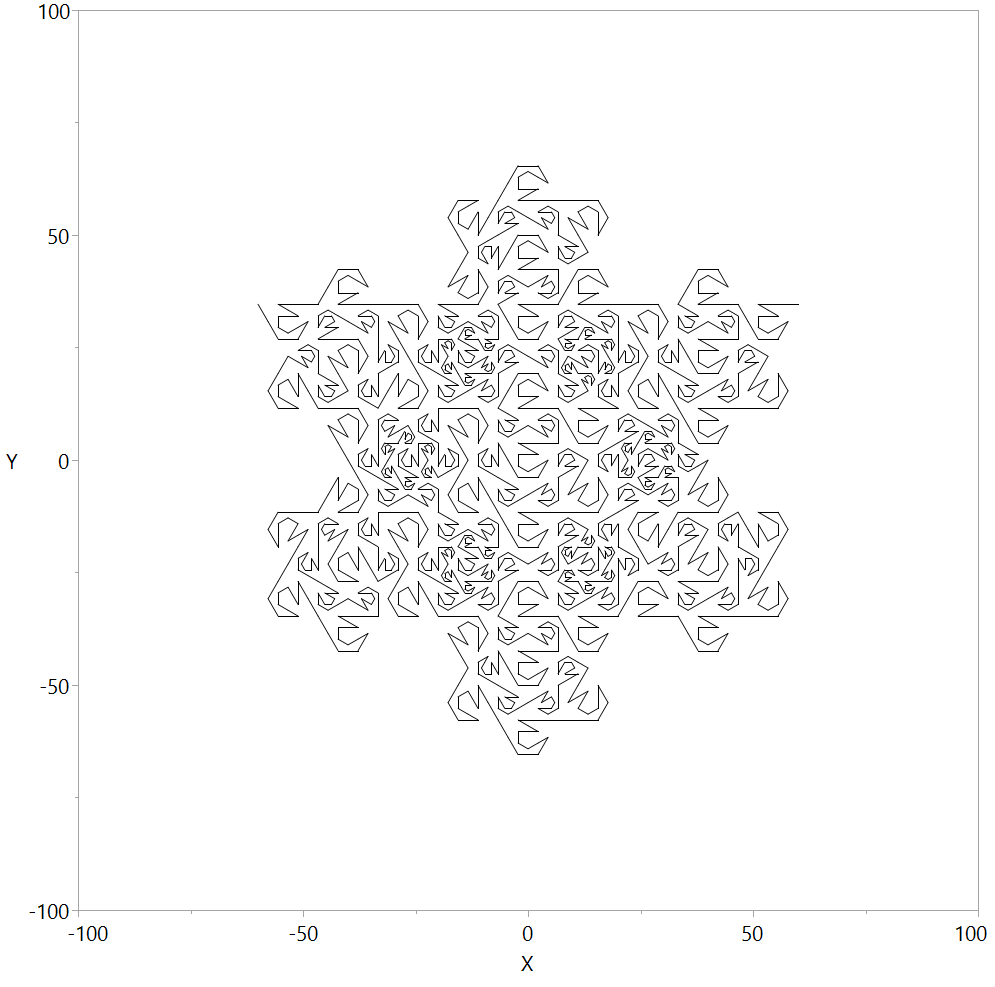Snowflake example

``````turtle:run( "Mandelbrot Peano Curve", // I first saw this here: https://www.scientificamerican.com/magazine/sa/1978/04-01/
Expr(
Local( {twist, S, F1, F3, M},
twist = Function( {sign, ss, aa, L},
turtle:turnRel( aa ); // this segment must turn and scale
turtle:scaleRel( ss ); // itself before drawing
L = L - 1; // then, depending on the recursion level
If( L > 0, // draw a smaller copy...
Recurse( -sign, F3, sign * 60, L );
Recurse( sign, F3, 0, L );
Recurse( sign, F3, sign * -60, L );
Recurse( sign, F3, sign * -60, L );
Recurse( sign, F1, sign * 210, L );
Recurse( -sign, F1, 0, L );
Recurse( -sign, F1, sign * 60, L );
Recurse( -sign, F1, sign * 60, L );
Recurse( sign, F3, sign * -270, L );
Recurse( sign, F1, sign * 210, L );
Recurse( -sign, F1, 0, L );
Recurse( -sign, F3, sign * -210, L );
Recurse( sign, F3, 0, L );
,   // else draw a straight line of length S at the current scale (ss)
turtle:walk( S )
);
// pop our scale, but NOT our angle...the angle keeps twisting about
turtle:scaleRel( 1 / ss );
);

S = 120;// size. this is adjustable.
// these are the scale-down amounts for the two smaller sizes that are used to
// replace a bigger one. thirteen smaller ones of one of these two sizes...
F1 = Sqrt( 1 + Tan( 30 * Pi() / 180 ) * Tan( 30 * Pi() / 180 ) ) / 6;
F3 = 2 / 6;

M = 4; // max depth. draws 13^(M-1) vectors, 4->2197, 5->28561, 6->371293, ...

turtle:moveAbs( -S / 2, (Tan( 30 * Pi() / 180 ) / 2) * S );// center at 0,0
twist( -1, 1, 0, M );
)
)
);``````

Tree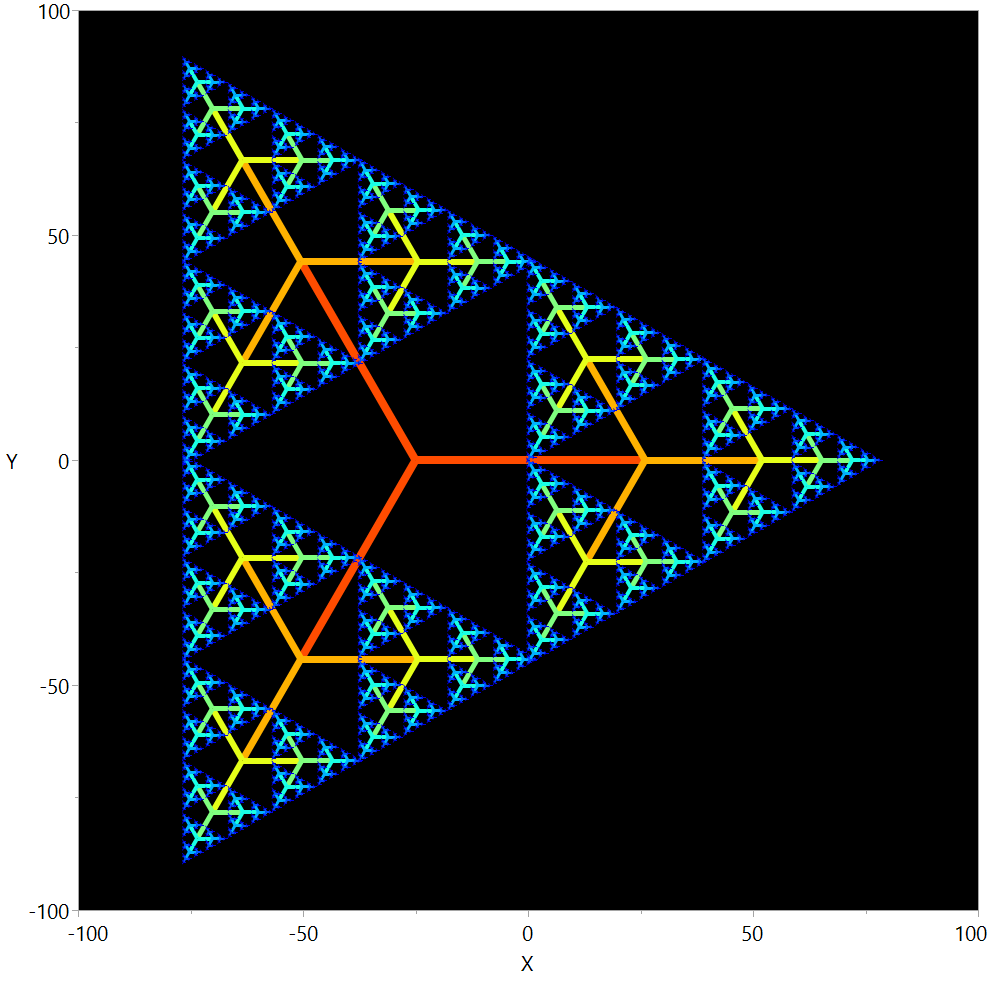Tree example (parameters tweaked for a triangle)

``````turtle:run( "Tree",
Expr(
Local( {tree, S, M},
tree = Function( {L}, {i, color, n, b = 3, f = .51},
turtle:scaleRel( f );
L = L - 1; // then, depending on the recursion level
If( L > 0, // draw a smaller copy...
color = Heat Color( L / (M + 1), "jet" );
n = 0;
For( i = 1, i <= b, i += 1,
turtle:turnRel( 360 / b );
If( Random Uniform() < .99999999, // make this .9 to randomly delete some bits
turtle:walk( S, color, L );
Recurse( L );
);
);
);
turtle:scaleRel( 1 / f );
);
S = 100;// size. this is adjustable.
M = 9;
turtle:moveAbs( -25, 0 );// for b==3
tree( M );
)
),
"black" // background color, default size
);``````

Rectangles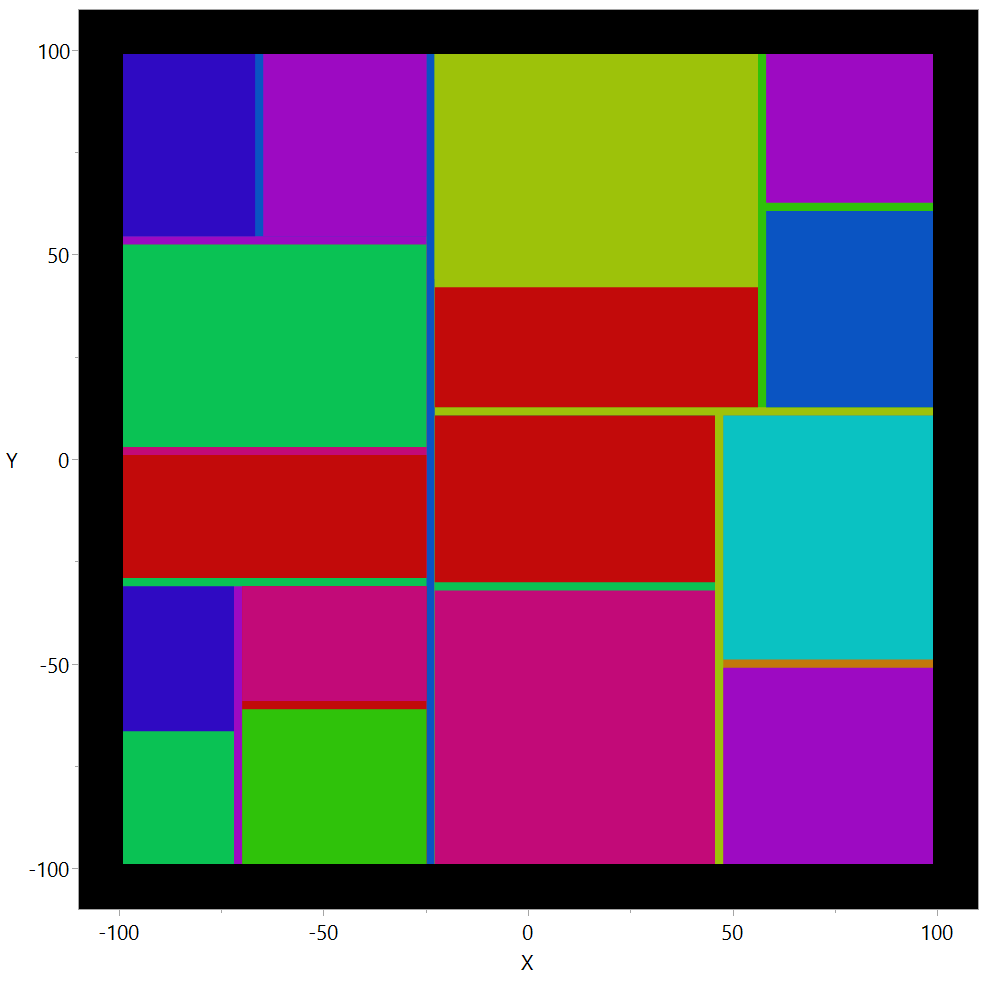Rectangle example

``````// using the 'hand' tool to drag this around will rerun the script an make a new random tiling each time
turtle:run( "Rectangular tiles",
Expr(
Local( {tile, M},
tile = Function( {top, left, bottom, right, L}, {i, color, hsplit, vsplit},
If( right - left > 3 & top - bottom > 3,
turtle:rectAbs( left + 1, top - 1, right - 1, bottom + 1, HLS Color( Random Integer( 0, 9 ) / 10, .4, .9 ) )
);
L = L - 1; // then, depending on the recursion level
If( L > 0 & (top - bottom > 7 & right - left > 7), // draw a smaller copy...
If( top - bottom > right - left, // separate top from bottom
vsplit = Random Uniform( bottom + (top - bottom) / 3, top - (top - bottom) / 3 );
Recurse( top, left, vsplit, right, L );
Recurse( vsplit, left, bottom, right, L );
, // separate left from right
hsplit = Random Uniform( left + (right - left) / 3, right - (right - left) / 3 );
Recurse( top, left, bottom, hsplit, L );
Recurse( top, hsplit, bottom, right, L );
)//
);
);
M = 5;
tile( 100, -100, -100, 100, M );
)
),
"black", 900, 900, -110, 110, -110, 110
);``````

Tiles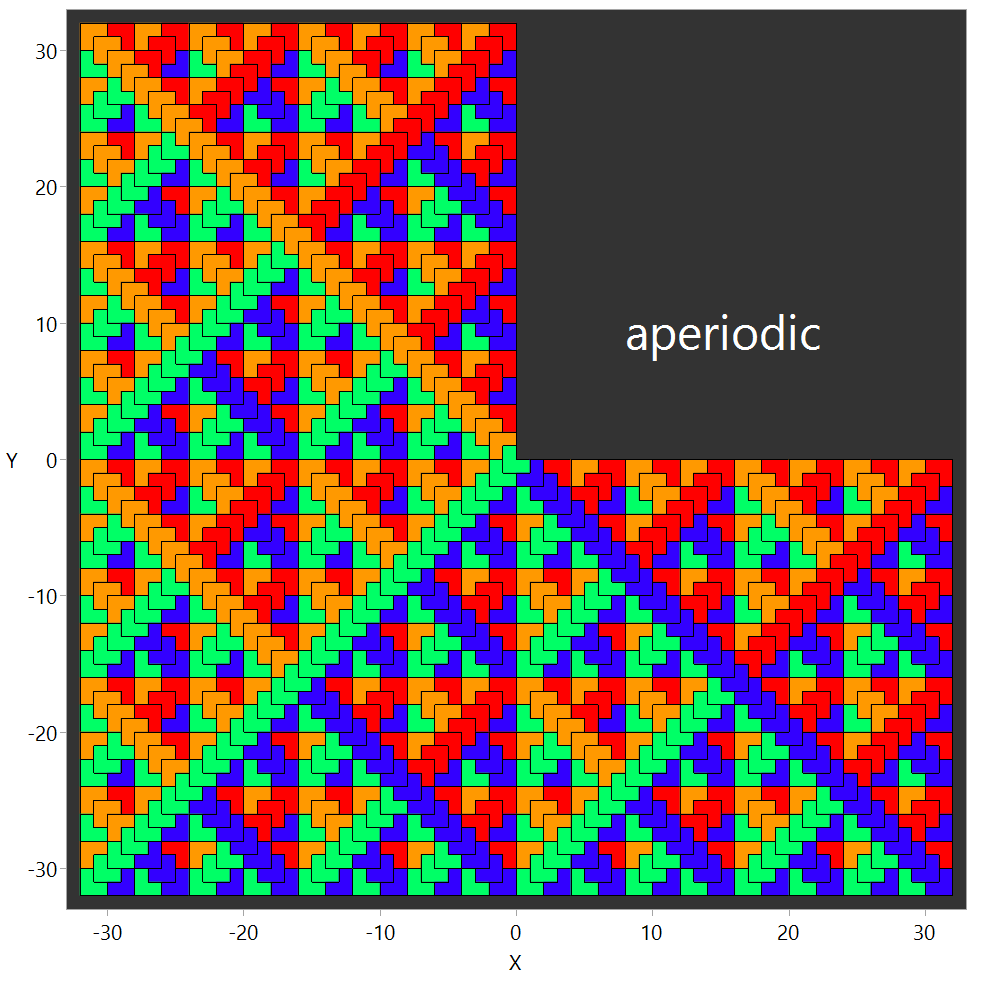Tile example

``````// aperiodic chair tile, http://paulbourke.net/geometry/tilingplane/ has the original idea

chairsize = 5; // 7 takes about 10 seconds. this is depth control and axis limit control
turtle:run(
"Chair tiles",
Expr(
Local( {chair},
CHAIR = Function( {insidex, insidey, m},
{x0, y0, x1, y1, x2, y2, x3, y3},
If( m == 0, // draw one tiny chair using angle to choose color
turtle:beginpath();
turtle:turnRel( 90 );
turtle:turnRel( 90 );
turtle:turnRel( 90 );
turtle:turnRel( 90 );
turtle:turnRel( 90 );
turtle:fillPath( HLS Color( turtle:angleDegrees / 300 + .1, .5, 1 ) );
turtle:outlinePath( "black" );
, // recursive: draw 4 chairs of the next smaller size
m -= 1;
CHAIR( insidex, insidey, m ); // 1 - inside corner
turtle:turnRel( 90 );
turtle:walk( 2 ^ m, "black", 0 );
turtle:turnRel( 90 );
turtle:walk( 2 ^ m, "black", 0 );
turtle:turnRel( -180 ); // the back corner chair is the same angle (90+90-180)
CHAIR( insidex, insidey, m ); // 2 - back corner
turtle:walk( 2 * 2 ^ m, "black", 0 );
turtle:turnRel( -90 ); // top corner is rotated -90
CHAIR( insidex, insidey, m ); // 3 -- top corner
turtle:walk( 2 * 2 ^ m, "black", 0 );
turtle:turnRel( -90 );
turtle:walk( 2 * 2 ^ m, "black", 0 );
turtle:turnRel( -90 ); // bottom corner is rotated +90 (-90-90-90)
CHAIR( insidex, insidey, m ); // 4 -- bottom corner
turtle:walk( 2 ^ m, "black", 0 ); // return to original angle and position
turtle:turnRel( -90 );
turtle:walk( 2 ^ m, "black", 0 );
)
);
turtle:turnAbs( 90 ); // absolute initial orientation
CHAIR( 0, 0, chairsize );
turtle:moveabs( 2 * (chairsize - 1), 2 * (chairsize - 1) );
turtle:say( "aperiodic", "white", 36 );
)
),
HLS Color( 0, .2, 0 ), 900, 900, -(2 ^ chairsize + 1), (2 ^ chairsize + 1), -(2 ^ chairsize + 1), (2 ^ chairsize + 1)
);``````

Article Labels

There are no labels assigned to this post.

Article Tags